# David Silver《Reinforcement Learning》课程解读—— Lecture 5： Model-Free Control

DP动态规划能够解决已知environment的MDP问题，即已知 S,A,P,R,γ$\ S,A,P,R,γ$，根据是否已知policy又将问题划分为prediction和control的问题。本质上来说这种known MDP问题已知environment即转移矩阵与reward函数

episode：从某个状态开始执行到终止状态的一次遍历S1,A1,R2,Sk$S_1,A_1,R_2,···，S_k$称为episode，已知很多episodes。

• Introduction
• On-Policy Monto-Carlo Control
• On-Policy Temporal-Difference
• Off-Policy Learning
• Summary

## 1. Introduction

1. 分类
1. Model-Free Prediction
estimate the value function of an unknown MDP，即已知policy求解未知environment的MDP问题。
2. Model-Free Control
optimize the value function of an unknown MDP，即未知policy求解未知environment的MDP问题。
2. Model-Free Control中获取policy的2中思路：
1. on-policy Learning：基于某个policy做出一些action然后评估其效果。
2. off-policy Learning：从已知policy中学习新的policy

## 2. On-policy Monte-Carlo Control

1. 组成 即一种新的策略迭代方法
policy evaluation + ϵ$\epsilon$-Greedy Policy Improvement

2. Generalised Policy Iteration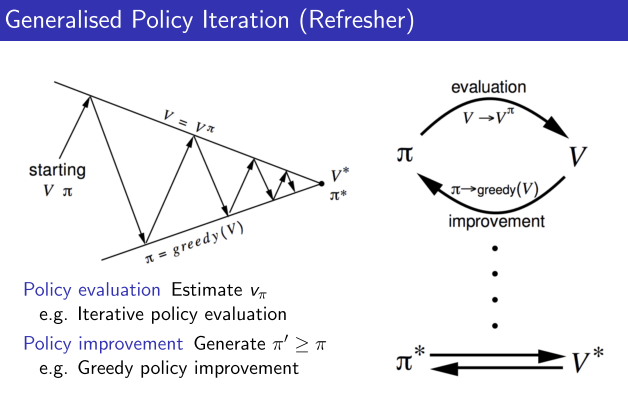1. Model-Free Policy Iteration with Monte-Carlo Evaluation
先通过贪婪算法来确定当前的policy，再通过蒙特卡洛policy evaluation来评估当前的policy好不好，再更新policy。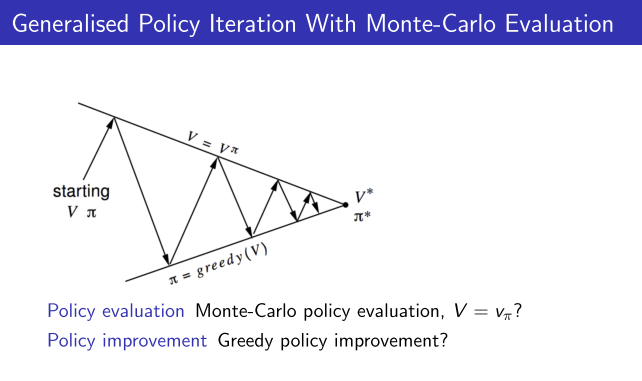2. Model-Free Policy Iteration using Action-Value Function
如果在已知environment情况下policy improvement更新方式是π=argmaxaARas+PassV(s)${\pi }'=\underset{a\in A}{argmax}R_{s}^{a}+P_{s{s}'}^{a}V\left ( {s}' \right )$，可以看出它的解决方案是通过状态转移矩阵把所有可能转移到的状态得到的值函数都计算出来，从中来选择最大的。但未知environment则没有状态转移矩阵，因此只能通过最大化动作值函数来更新policy即π(s)=argmaxaAQ(s,a)${\pi }'\left ( s \right )=\underset{a\in A}{argmax}Q\left ( s,a\right )$。由于improvement的过程需要动作值函数，那么在policy evaluation的过程中针对给定的policy需要计算的V(s)$V\left ( s \right )$也替换成Q(s,a)$Q\left ( s,a\right )$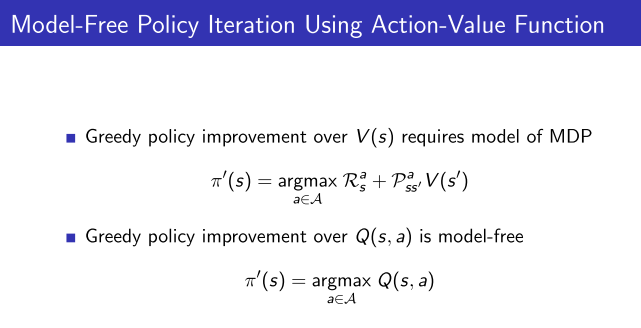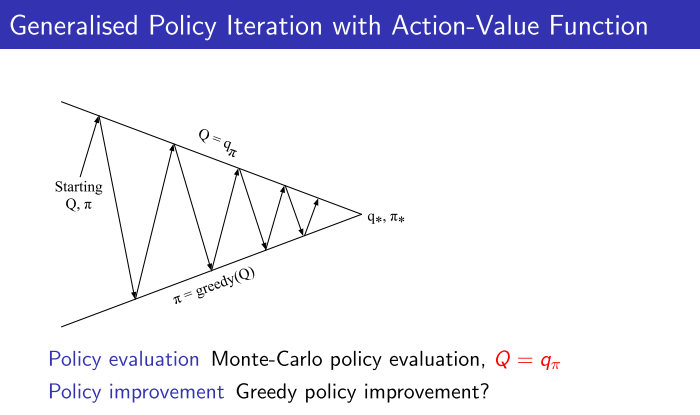3. ϵ$\epsilon$-Greedy Exploration
常规策略迭代的改进算法，在上面greedy基础上有一定概率ϵ$\epsilon$地选择一个随机action。假设有m个action，那么有ϵ$ϵ$的概率随机选择一个action(包括greedy action)，从而可以得到更新的policy为:
4. 12
由于每一步的reward都知道，则意味着每一步的return Gt$G_{t}$都可以计算出来。因此，通过反复测试，这样很多状态就会被遍历到，而且不止一次，那么每次就可以把在状态下的return求取平均值。当episode无限大时，得到的数据也就接近于真实的数据。Monte-Carlo方法就是使用统计学的方法来取代Bellman方法的计算方法。
- 有两种访问次数的记录方式，一种是在一个episode中只记录第一次访问到的s，一种是一个episode中每次访问到s都记录下来。
- First-Visit MC Policy Evaluation，每一次的episode中state只使用第一次到达的t来计算return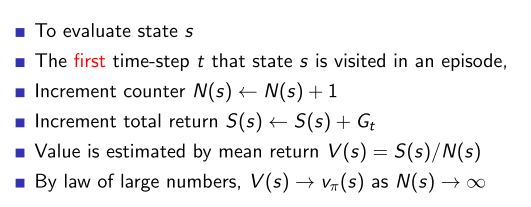- Every-Visit MC Policy Evaluation，每一次的episode中state只要访问到就计算return求平均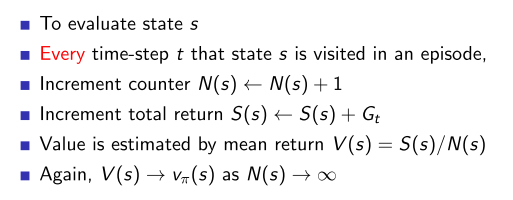• 特点：可以看到蒙特卡洛方法是极其简单的。但是缺点也是很明显的，需要尽可能多的反复测试，而且需要到每一次测试结束后才来计算，需要耗费大量时间。“AlphaGo就是使用蒙特卡洛的思想。不是蒙特卡洛树搜索，而是说在增强学习中使用蒙特卡洛方法的思想。AlphaGo每次也是到下棋结束，而且只使用最后的输赢作为return。所以这也是非常神奇的事，只使用最后的输赢结果，竟然能够优化每一步的走法。”

• Incremental Monte-Carlo

• Incremental Mean
序列{x1,x2,}$\left \{ x_{1},x_{2},\cdot \cdot \cdot \right \}$的均值{u1,u2,}$\left \{ u_{1},u_{2},\cdot \cdot \cdot \right \}$可以增量式增加

uk====1kj=1kxj1kxk+j=1k1xj1k(xk+(k1)uk1)uk1+1k(xkuk1)

• Updates V(s) incrementally after episode S1,A1,R2,,ST$S_1, A_1, R_2, ···,S_T$
• 针对每一次新的访问，先次数加1，N(St)=N(St)+1$N(S_t)=N(S_t)+1$，然后更新V(St)=V(St)+1N(St)(GtV(St))$V(S_t)=V(S_t)+\frac{1}{N(S_t)}(G_t-V(S_t))$
• 在解非固定问题时，可以将1N(St)$\frac{1}{N(S_t)}$设置为一个常数α$\alpha$，即V(St)=V(St)+α(GtV(St))$V(S_t)=V(S_t)+\alpha(G_t-V(S_t))$.

## 3. Temporal-Difference Learning

1. 思想
基于Bootstrapping思想，即在中间状态中会估计当前获得的return值，并更新之前状态能获得的return，因此它不需要走完一个episode的全部流程而前面分析的蒙特卡洛方法，其一个特点就是需要运行完整个episode从而获得准确的result。但是往往很多场景下要运行完整个episode是很费时间的
2. 特点
• 直接从experience的episode中学习
• 不需要MDP的transitions、rewards
• 通过bootstrapping从不完整的episode中学习
bootstraping。即通过估计的方法来引导计算。那么蒙特卡罗不使用bootstraping，而TD使用bootstraping。
• 每一步都可以更新，这是显然，也就是online learning，学习快
• 从一个猜测更新到另一个猜测
• 可以面对没有结果的场景，应用范围广
3. TD vs MC
• 目标：learn vπ$v_\pi$ online from experience under policy π$\pi$.
• Incremental every-visit Monte-Carlo
根据真实的 reward Gt$G_t$更新 V(St)$V(S_t)$:
V(St)=V(St)+α(GtV(St))
• Simplest temporal-difference learning algorithm: TD(0)
根据估计的return更新V(st)$V(s_t)$, 估计return：Rt+1+γV(St+1)$R_{t+1}+\gamma V(S_t+1)$:
V(St)=V(St)+α(Rt+1+γV(St+1)V(St))

其中，Rt+1+γV(St+1)$R_{t+1}+\gamma V(S_t+1)$TD target,
δt=Rt+1+γV(St+1)V(St)$\delta _{t}=R_{t+1}+\gamma V(S_t+1)-V(S_t)$TD error, 代表了估计前后return的差值
• 平衡Bias/Variance是机器学习比较经典的一个问题，bias是指预测结果与真实结果的差值，variance是指训练集每次预测结果之间的差值，bias过大会导致欠拟合它衡量了模型是否准确，variance过大会导致过拟合衡量了模型是否稳定。
• TD算法的相对缺点：因为TD target是估计值，估计是有误差的，这就会导致更新得到value是有偏差的。很难做到无偏估计。
• TD算法的相对优点：TD target 是每一个 step 进行估计的，仅最近的动作对其有影响，而 MC 的 result 则受到整个时间片中动作的影响，因此 TD target 的方差 variance 会比较低，也就是波动性小。
• 具体对比如下图所示：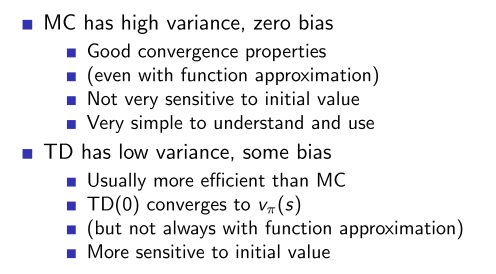• 在David Silver的课件中，有三张图，很直观地对比了MC，TD以及DP的不同：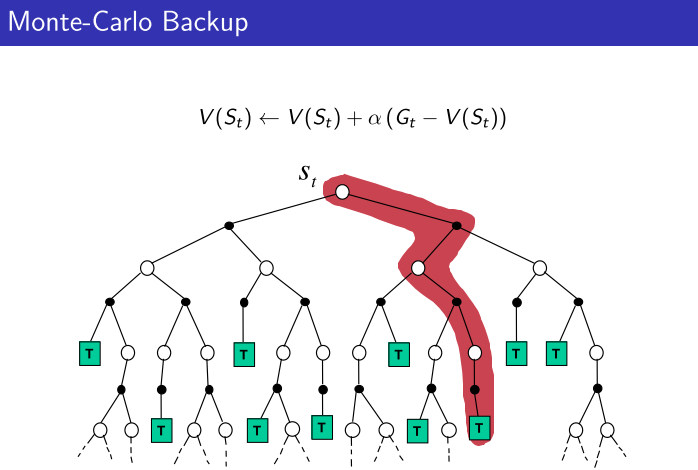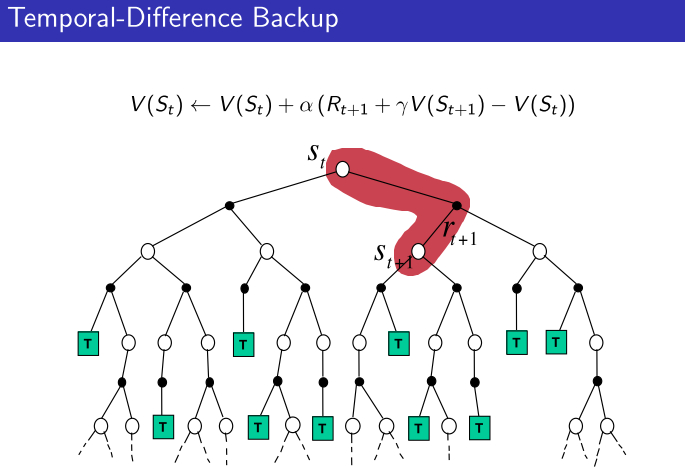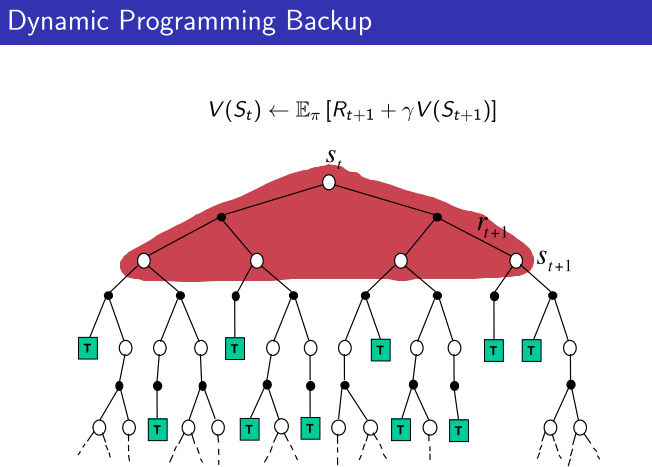从上面可以很清楚的看到三者的不同。DP就是理想化的情况，遍历所有。MC现实一点，TD最现实，但是TD也最不准确。但是没关系，反复迭代之下，还是可以收敛的。
• 整个增强学习算法也都在上面的范畴里：• 上面的图是用Policy Evaluation解决强化学习问题的一些算法的区别与相关性，最左边的竖线表示如果考虑了所有的可能发生的情况那么就是动态规划，如果只考虑了部分采样那么就是时序差分。下面的横线表示如果考虑了一次episode中全部的动作就是Monte-Carlo，如果只考虑部分动作就是时序差分。如果又考虑全部情况又考虑每一种情况的全部动作就是穷举。

## 4. TD(λ)$\left ( \lambda \right )$

1. 思想
• TD(0)$TD\left ( 0 \right )$: 在某个状态S$S$下执行某个动作后转移到下一个状态S${S}'$时，估计S${S}'$的return再更新S。
• S$S$之后执行2次动作转移到S′′${S}''$时再返回来更新S$S$的值函数，那么就是另外一种形式，从而根据step的长度n可以扩展TD到不同形式，当step的长度达到episode的终点时就变成了MC，从而得到统一公式如下：
Gn=Rt+1+γRt+2++γn1Rt+n+rnV(St+n)

V(St)=V(St)+α(G(n)tV(St))

• TD(λ)$TD\left ( \lambda \right )$: 若将不同的n对应的return平均一下，就能获得更加鲁邦的结果，而为了有效地将不同return结合起来，对每个return都赋予了一个权重1λ,(1λ)lambda,,(1λ)λn$1-\lambda,\left ( 1-\lambda \right )lambda,\cdot \cdot \cdot ,\left ( 1-\lambda \right )\lambda^{n}$，参数是lambda$lambda$，这样又可以得到一组更新value function公式：
Gλt=(1λ)n=1λn1Gnt

V(St)=V(St)+α(GλtV(St))©️2019 CSDN 皮肤主题: 大白 设计师: CSDN官方博客